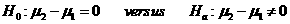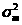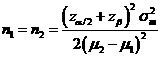# Sample Size Calculator: Two Crossover-Sample Means

Hypothesis: Two-Sided EqualityData Input: (Help) (Example)

 Input Results α β n Allowable difference Population variance

Note:

 Variables Descriptions α Two-sided significance level 1-β Power of the test Allowable difference Acceptable mean difference between sample two and sample one (µ2-µ1) Population variance Population variancen Sample size of each group

Help Aids

Application: Consider a 2×2 cross-over design contains two sequences (treatment orderings) and two time periods (occasions). One sequence receives treatment A followed by treatment B. The other sequence receives B and then A. This procedure is used to test the following hypotheses:Procedure:

1. Enter

a)      value of α, the probability of type I error

b)      value of β, the probability of type II error

c)      value of allowable difference

d)     value of population variance

1. Click the button “Calculate” to obtain result sample size of each group n.

Formula:(*)

Notations:

α:               The probability of type I error (significance level) is the probability of rejecting the true null hypothesis.

β:               The probability of type II error (1 – power of the test) is the probability of failing to reject the false null hypothesis.

μ2 – μ1:      The value of allowable difference is the true mean difference between a test drugs (μ2) and a placebo control or active control agent (μ1).

Examples

Example 1: Suppose a low density lipidproteins (LDLs) is considered of clinically meaningful difference. By using (*), assuming that the standard deviation is 10% (i.e., population variance is 0.01), the required sample size of each group to achieve an 80% power (β=0.2) at α=0.05 for correctly detecting such difference of μ2 – μ1=0.5 change obtained by normal approximation as n=16.

Reference: Chow, Shao and Wang, Sample Size Calculations In Clinical Research, Taylor & Francis, NY. (2003) Pages 64-65.